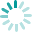## XOXO

https://www.knittingunlimited.com/2022/06/blanket-31-hugs-and-kisses.html

Shown with a cast-on count of 45 stitches.• Row 1 (RS): [K1, p1] 3 times, [p4, k2tog, k2, yo, p1, yo, k2, ssk, p3] twice, p2, [k1, p1] twice, k1.
• Rows 2 and 30: [K1, p1] 3 times, k1, [k3, p4, k1, p4, k4] twice, [p1, k1] 3 times.
• Row 3: [K1, p1] 3 times, [p3, k2tog, k2, yo, k1, p1, k1, yo, k2, ssk, p2] twice, p2, [k1, p1] twice, k1.
• Rows 4 and 28: [K1, p1] 3 times, k1, [k2, p5, k1, p5, k3] twice, [p1, k1] 3 times.
• Row 5: [K1, p1] 3 times, [p2, k2tog, k2, yo, k2, p1, k2, yo, k2, ssk, p1] twice, p2, [k1, p1] twice, k1.
• Rows 6 and 26: [K1, p1] 3 times, k1, [k1, p6, k1, p6, k2] twice, [p1, k1] 3 times.
• Row 7: [K1, p1] 3 times, [p1, k2tog, k2, yo, k3, p1, k3, yo, k2, ssk] twice, p2, [k1, p1] twice, k1.
• Rows 8 and 24: [K1, p1] 3 times, k1, [p7, k1, p7, k1] twice, [p1, k1] 3 times.
• Row 9: [K1, p1] 3 times, [p1, k3, k2tog, k2, yo, p1, yo, k2, ssk, k3] twice, p2, [k1, p1] twice, k1.
• Rows 10 and 22: [K1, p1] 3 times, k1, [p6, k3, p6, k1] twice, [p1, k1] 3 times.
• Row 11: [K1, p1] 3 times, [p1, k2, k2tog, k2, yo, p3, yo, k2, ssk, k2] twice, p2, [k1, p1] twice, k1.
• Row 12: [K1, p1] 3 times, k1, [p5, k5, p5, k1] twice, [p1, k1] 3 times.
• Row 13: [K1, p1] 3 times, [p1, k1, k2tog, k2, yo, p5, yo, k2, ssk, k1] twice, p2, [k1, p1] twice, k1.
• Row 14: [K1, p1] 3 times, k1, [p4, k7, p4, k1] twice, [p1, k1] 3 times.
• Row 15: [K1, p1] 3 times, [p1, k2tog, k2, yo, p7, yo, k2, ssk] twice, p2, [k1, p1] twice, k1.
• Row 16: [K1, p1] 3 times, k1, [p3, k9, p3, k1] twice, [p1, k1] 3 times.
• Row 17: [K1, p1] 3 times, [p1, yo, k2, ssk, p7, k2tog, k2, yo] twice, p2, [k1, p1] twice, k1.
• Row 18: [K1, p1] 3 times, k1, [p4, k6, p5, k1] twice, [p1, k1] 3 times.
• Row 19: [K1, p1] 3 times, [p1, k1, yo, k2, ssk, p5, k2tog, k2, yo, k1] twice, p2, [k1, p1] twice, k1.
• Row 20: [K1, p1] 3 times, k1, [p5, k4, p6, k1] twice, [p1, k1] 3 times.
• Row 21: [K1, p1] 3 times, [p1, k2, yo, k2, ssk, p3, k2tog, k2, yo, k2] twice, p2, [k1, p1] twice, k1.
• Row 23: [K1, p1] 3 times, [p1, k3, yo, k2, ssk, p1, k2tog, k2, yo, k3] twice, p2, [k1, p1] twice, k1.
• Row 25: [K1, p1] 3 times, [p1, yo, k2, ssk, k3, p1, k3, k2tog, k2, yo] twice, p2, [k1, p1] twice, k1.
• Row 27: [K1, p1] 3 times, [p2, yo, k2, ssk, k2, p1, k2, k2tog, k2, yo, p1] twice, p2, [k1, p1] twice, k1.
• Row 29: [K1, p1] 3 times, [p3, yo, k2, ssk, k1, p1, k1, k2tog, k2, yo, p2] twice, p2, [k1, p1] twice, k1.
• Row 31: [K1, p1] 3 times, [p4, yo, k2, ssk, p1, k2tog, k2, yo, p3] twice, p2, [k1, p1] twice, k1.
• Row 32: [K1, p1] 3 times, k1, [k4, p3, k1, p3, k5] twice, [p1, k1] 3 times.
• Repeat rows 1-32.